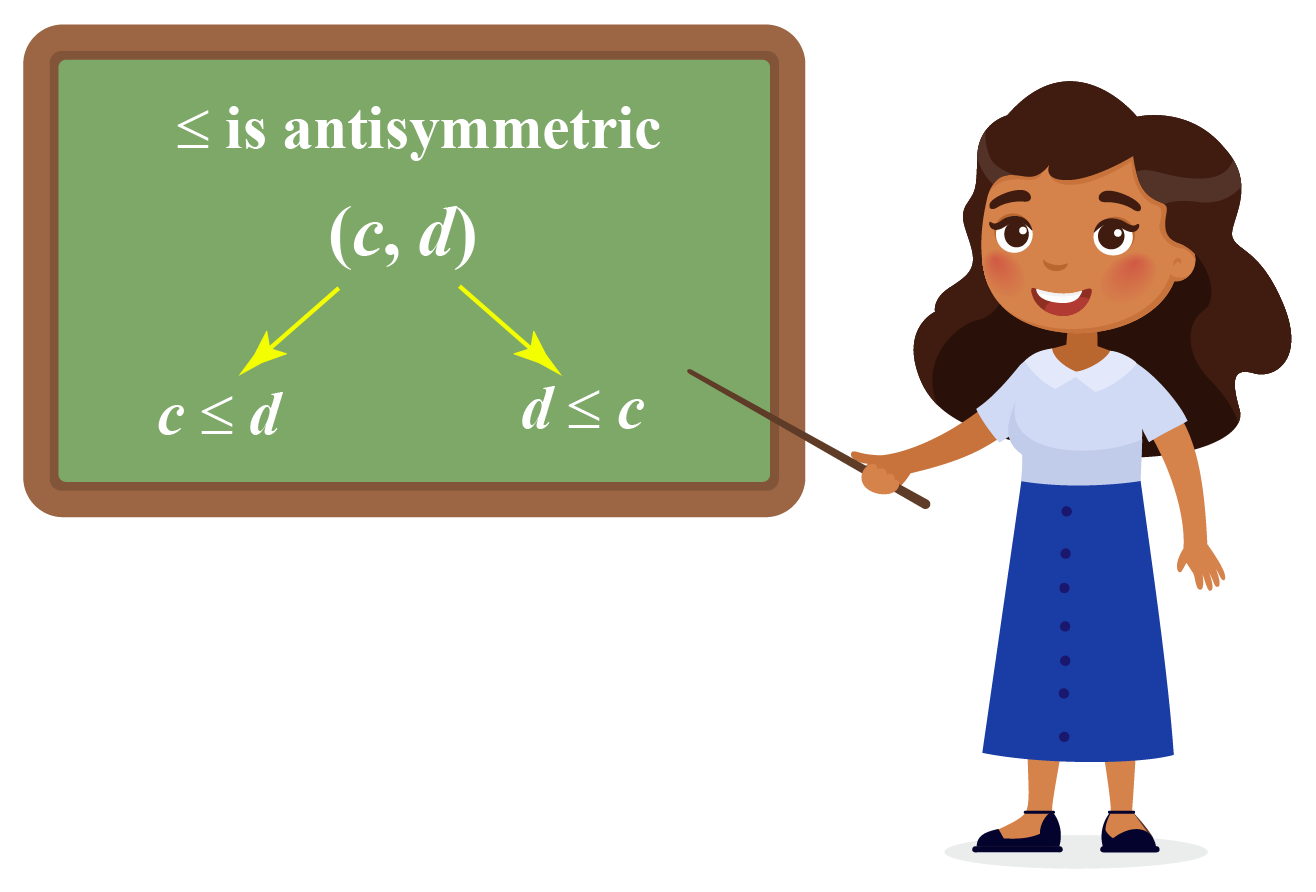# Antisymmetric Relation

Antisymmetric Relation

"What is an antisymmetric relation?" asked Miss Granger in the geometry class.

Nicole, who had always loved discrete mathematics said, "A relation in which an element 'a' is related to another element 'b' and 'b' is related to 'a' only if a=b."

When you ask someone the question: 'What is an antisymmetric relation?' then Nicole's answer is almost perfect.

In this section, we’re going to look at the antisymmetric relation examples and antisymmetric relations.## Lesson Plan

 1 What Is Meant By Antisymmetric Relation? 2 Important Notes on Antisymmetric Relation 3 Solved Examples on Antisymmetric Relation 4 Interactive Questions on Atisymmetric Relation 5 Think Out of the Box!

## What Is Meant By Antisymmetric Relation?

Let us first recall the meaning of a relation.

A relation is just a collection of ordered pairs.

The first element n in the ordered pair is related to the second element according to the relation defined.

The set of input numbers is called the domain of the relation, and the set of output numbers is called the range of the relation.

Let us now understand the meaning of antisymmetric relations.

A relation R on a set A is said to be antisymmetric if there does not exist any pair of distinct elements of A which are related to each other by R.

Mathematically, it is denoted as:

 For all a, b $$\in$$ A, If (a,b) $$\in$$ R and (b,a) $$\in$$ R, then a=b

Equivalently,

For all a, b $$\in$$ A,

If (a,b) $$\in$$ R and a $$\neq$$ b, then (b,a) $$\in$$ R must not hold.

## Where Can You Use the Concept of Antisymmetric Relation?

Let us explore some examples where antisymmetric relations can be used.

### Example

Define a relation R on a set X as:

An element $$x$$ in X is related to an element $$y$$ in X as $$x$$ is divisible by $$y$$.

That is, $$(x,y) \in$$ R if and only if $$x$$ is divisible by $$y$$

We will determine if R is an antisymmetric relation or not.

Assume $$(x,y) \in R$$ and $$(y,x) \in R$$.

This implies $$x$$ is divisible by $$y$$ and $$y$$ is divisible by $$x$$

This is possible only if $$x=y$$

To verify this, let us consider particular values of $$x$$ and $$y$$

Suppose $$x=4$$ and $$y=2$$

We can say that (4,2) $$\in$$ R but (2,4) $$\notin$$ R because 4 is divisible by 2 but 2 is not divisible by 4

Hence, R is an antisymmetric relation.

More Important Topics
Numbers
Algebra
Geometry
Measurement
Money
Data
Trigonometry
Calculus
More Important Topics
Numbers
Algebra
Geometry
Measurement
Money
Data
Trigonometry
Calculus
Learn from the best math teachers and top your exams

• Live one on one classroom and doubt clearing
• Practice worksheets in and after class for conceptual clarity
• Personalized curriculum to keep up with school